# Pandas & Numpy (Space Stone of Programming World)

Today, we’ll demonstrate the different application of Pandas. In this case, we’ll be exploring the possibilities of reading large CSV files & splitting it sets of smaller more manageable csv to read.

And, after creating it, another process will merge them together. This is especially very useful when you need transformation on a large volume of data without going for any kind of memory error. And, moreover, the developer has more control over failed cases & can resume the load without restarting it from the beginning of the files.

In this case, I’ll be using one more custom methods to create the csv file instead of directly using the to_csv method of pandas.

But, before that let’s prepare the virtual environment & proceed from there –

Windows 10 (64 bit):

##### Commands:

python -m venv –copies .env

.env\Scripts\activate.bat

##### Screenshot:Mac OS (64 bit):

##### Commands:

python -m venv env

source env/bin/activate

##### Screenshot:So, both the Windows & Mac version is 3.7 & we’re going to explore our task in the given section.

After creating this virtual environment, you need to install only pandas package for this task as shown below for both the Windows or Mac OS –

##### Windows: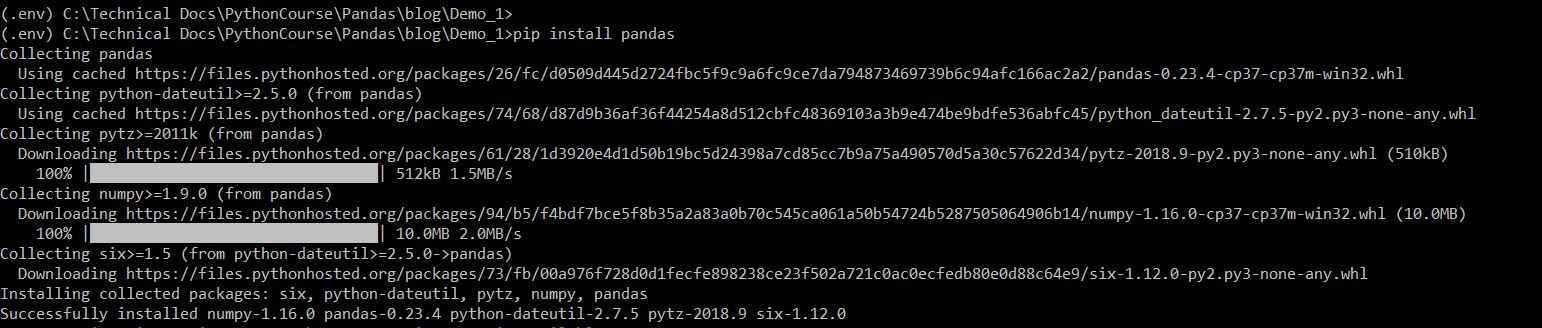##### Mac: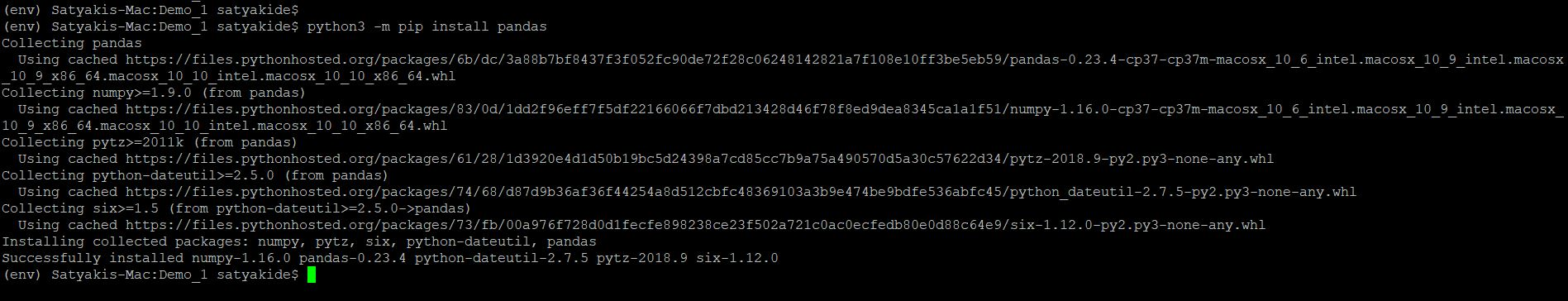Rests are the packages comes as default with the Python 3.7.

Please find the GUI screenshots from WinSCP software comparing both the directory structures (Mac & Windows) as given below –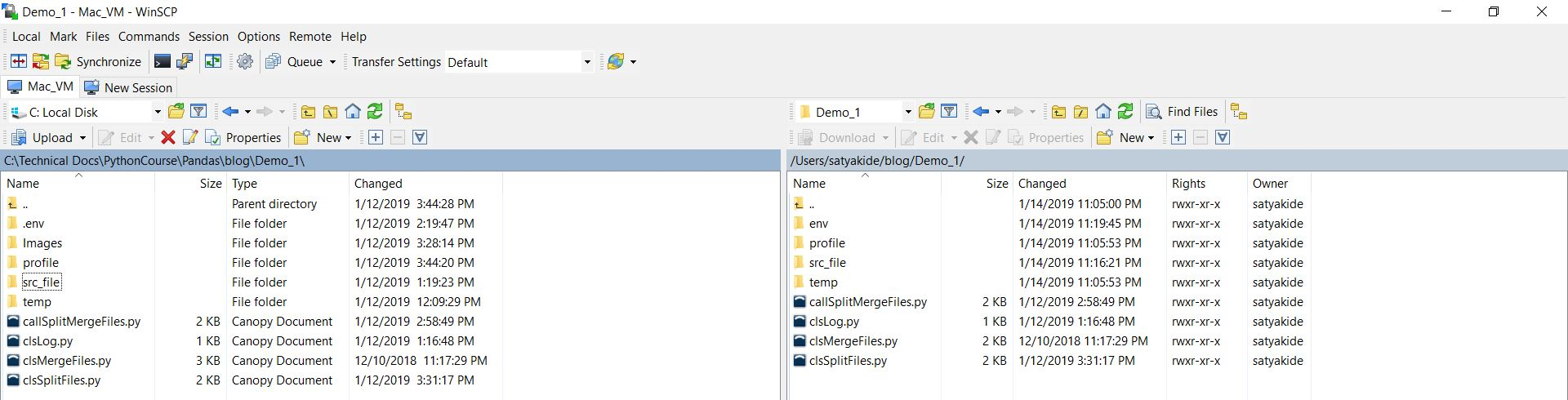From the above screenshot, you can see that our directory structure are not exactly identical before the blog directory. However, our program will take care of this difference.

Let’s check the scripts one-by-one,

1. clsL.py (This script will create the split csv files or final merge file after the corresponding process. However, this can be used as normal verbose debug logging as well. Hence, the name comes into the picture.)

 ``` 1 2 3 4 5 6 7 8 9 10 11 12 13 14 15 16 17 18 19 20 21 22 23 24 25 26 27 28 29 30 31 32 33 34 35 36 37 38 39 40``` ```############################################# #### Written By: Satyaki De #### ############################################# import pandas as p import os import platform as pl class clsL(object): def __init__(self): self.path = os.path.dirname(os.path.realpath(__file__)) def logr(self, Filename, Ind, df, subdir=None): try: x = p.DataFrame() x = df sd = subdir os_det = pl.system() if os_det == "Windows": if sd == None: fullFileName = self.path + "\\" + Filename else: fullFileName = self.path + "\\" + sd + "\\" + Filename else: if sd == None: fullFileName = self.path + "/" + Filename else: fullFileName = self.path + "/" + sd + "/" + Filename if Ind == 'Y': x.to_csv(fullFileName, index=False) return 0 except Exception as e: y = str(e) print(y) return 3 ```

From the above script, you can see that based on the Indicator, whose value can be either ‘Y’ or ‘N’. It will generate the csv file from the pandas data frame using to_csv method available in pandas.

Key snippet to notice –

self.path = os.path.dirname(os.path.realpath(__file__))

Here, the class is creating an instance & during that time it is initializing the value of the current path from where the application is triggering.

x = p.DataFrame()
x = df

The first line, declaring a pandas data frame variable. The second line assigns the value from the supplied method to that variable.

os_det = pl.system()

This will identify the operating system on which your application is running. Based on that, your path will be dynamically configured & passed. Hence, your application will be ready to handle multiple operating systems since beginning.

x.to_csv(fullFileName, index=False)

Finally, to_csv will generate the final csv file based on the supplied Indicator value. Also, notice that we’ve added one more parameter (index=False). By default, pandas create one extra column known as an index & maintain it’s operation based on that.As you can see that the first column is not coming from our source files. Rather, it is generated by the pandas package in python. Hence, we don’t want to capture that in our final file by mentioning (index=False) options.

2. clsSplitFl.py (This script will create the split csv files. This will bring chunk by chunk data into your memory & process the large files.)

 ``` 1 2 3 4 5 6 7 8 9 10 11 12 13 14 15 16 17 18 19 20 21 22 23 24 25 26 27 28 29 30 31 32 33 34 35 36 37 38 39 40 41 42 43 44 45 46 47 48 49 50 51 52 53 54 55 56 57 58 59 60 61 62 63 64 65 66 67``` ```############################################# #### Written By: Satyaki De #### ############################################# import os import pandas as p import clsLog as log import gc import csv class clsSplitFl(object): def __init__(self, srcFileName, path, subdir): self.srcFileName = srcFileName self.path = path self.subdir = subdir # Maximum Number of rows in CSV # in order to avoid Memory Error self.max_num_rows = 30000 self.networked_directory = 'src_file' self.Ind = 'Y' def split_files(self): try: src_dir = self.path subdir = self.subdir networked_directory = self.networked_directory # Initiate Logging Instances clog = log.clsLog() # Setting up values srcFileName = self.srcFileName First_part, Last_part = str(srcFileName).split(".") num_rows = self.max_num_rows dest_path = self.path remote_src_path = src_dir + networked_directory Ind = self.Ind interval = num_rows # Changing work directory location to source file # directory at remote server os.chdir(remote_src_path) src_fil_itr_no = 1 # Split logic here for df2 in p.read_csv(srcFileName, index_col=False, error_bad_lines=False, chunksize=interval): # Changing the target directory path os.chdir(dest_path) # Calling custom file generation method # to generate splitted files clog.logr(str(src_fil_itr_no) + '__' + First_part + '_' + '_splitted_.' + Last_part, Ind, df2, subdir) del [[df2]] gc.collect() src_fil_itr_no += 1 return 0 except Exception as e: x = str(e) print(x) return 1 ```

In this script, we’re splitting the file if that file has more than 30,000 records. And, based on that it will split a number of equal or fewer volume files.

Important lines to be noticed –

self.max_num_rows = 30000

As already explained, based on this the split files contain the maximum number of rows in each file.

First_part, Last_part = str(srcFileName).split(“.”)

This will split the source file name into the first part & second part i.e. one part contains only the file name & the other part contains only the extension dynamically.

As you can see, the chunk-by-chunk (mentioned as chunksize=interval) application will read lines from the large source csv. And, if it has any bad rows in the source files – it will skip them due to the following condition -> (error_bad_lines=False).

clog.logr(str(src_fil_itr_no) + ‘__’ + First_part + ‘_’ + ‘_splitted_.’ + Last_part, Ind, df2, subdir)

Dynamically generating split files in the specific subdirectory along with the modified name. So, these files won’t get overwritten – if you rerun it. Remember that the src_fil_itr_no will play an important role while merging them back to one as this is a number representing the current file’s split number.

del [[df2]]
gc.collect()

Once, you process that part – delete the data frame & deallocate the memory. So, that you won’t encounter any memory error or a similar issue.

And, the split file will look like this –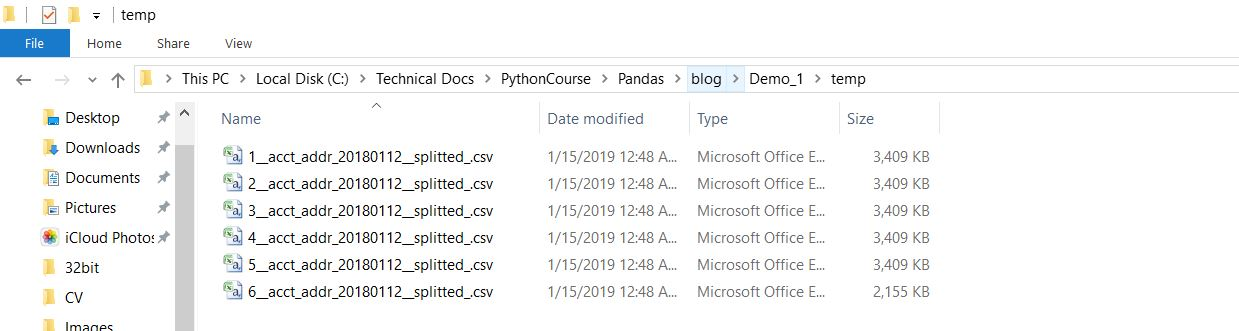3. clsMergeFl.py (This script will add together all the split csv files into one big csv file. This will bring chunk by chunk data into your memory & generates the large file.)

 ``` 1 2 3 4 5 6 7 8 9 10 11 12 13 14 15 16 17 18 19 20 21 22 23 24 25 26 27 28 29 30 31 32 33 34 35 36 37 38 39 40 41 42 43 44 45 46 47 48 49 50 51 52 53 54 55 56 57 58 59 60 61 62 63 64 65 66 67 68 69 70 71 72 73 74 75 76 77 78 79 80 81 82``` ```############################################# #### Written By: Satyaki De #### ############################################# import os import platform as pl import pandas as p import gc import clsLog as log import re class clsMergeFl(object): def __init__(self, srcFilename): self.srcFilename = srcFilename self.subdir = 'finished' self.Ind = 'Y' def merge_file(self): try: # Initiating Logging Instances clog = log.clsLog() df_W = p.DataFrame() df_M = p.DataFrame() f = {} subdir = self.subdir srcFilename = self.srcFilename Ind = self.Ind cnt = 0 os_det = pl.system() if os_det == "Windows": proc_dir = "\\temp\\" gen_dir = "\\process\\" else: proc_dir = "/temp/" gen_dir = "/process/" # Current Directory where application presents path = os.path.dirname(os.path.realpath(__file__)) + proc_dir print("Path: ", path) print("Source File Initial Name: ", srcFilename) for fname in os.listdir(path): if fname.__contains__(srcFilename) and fname.endswith('_splitted_.csv'): key = int(re.split('__', str(fname))) f[key] = str(fname) for k in sorted(f): print(k) print(f[k]) print("-"*30) df_W = p.read_csv(path+f[k], index_col=False) if cnt == 0: df_M = df_W else: d_frames = [df_M, df_W] df_M = p.concat(d_frames) cnt += 1 print("-"*30) print("Total Records in this Iteration: ", df_M.shape) FtgtFileName = fname.replace('_splitted_', '') first, FinalFileName = re.split("__", FtgtFileName) clog.logr(FinalFileName, Ind, df_M, gen_dir) del [[df_W], [df_M]] gc.collect() return 0 except Exception as e: x = str(e) print(x) return 1 ```

In this script, we’re merging smaller files into a large file. Following are the key snippet that we’ll explore –

```for fname in os.listdir(path):
if fname.__contains__(srcFilename) and fname.endswith('_splitted_.csv'):
key = int(re.split('__', str(fname)))
f[key] = str(fname)```

In this section, the application will check if in that specified path we’ve files whose extension ends with “_splitted_.csv” & their first name starts with the file name initial i.e. if you have a source file named – acct_addr_20180112.csv, then it will check the first name should start with the -> “acct_addr” & last part should contain “_splitted_.csv”. If it is available, then it will start the merge process by considering one by one file & merging them using pandas data frame (marked in purple color) as shown below –

```for k in sorted(f):
print(k)
print(f[k])
print("-"*30)

if cnt == 0:
df_M = df_W
else:
d_frames = [df_M, df_W]
df_M = p.concat(d_frames)

cnt += 1```

Note that, here f is a dictionary that contains filename in key, value pair. The first part of the split file contains the number.  That way, it would be easier for the merge to club them back to one large file without thinking of orders.

Here, also notice the special function concat provided by the pandas. In this step, applications are merging two data frames.

Finally, the main python script, from where we’ll call it –

4. callSplitMergeFl.py

 ``` 1 2 3 4 5 6 7 8 9 10 11 12 13 14 15 16 17 18 19 20 21 22 23 24 25 26 27 28 29 30 31 32 33 34 35 36 37 38 39 40 41 42 43 44 45 46 47 48 49 50 51 52 53 54 55 56 57 58 59 60 61 62``` ```############################################# #### Written By: Satyaki De #### ############################################# import clsSplitFl as t import clsMergeFl as cm import re import platform as pl import os def main(): print("Calling the custom Package for large file splitting..") os_det = pl.system() print("Running on :", os_det) ############################################################### ###### User Input based on Windows OS ######## ############################################################### srcF = str(input("Please enter the file name with extension:")) base_name = re.sub(r'[0-9]','', srcF) srcFileInit = base_name[:-5] if os_det == "Windows": subdir = "\\temp\\" path = os.path.dirname(os.path.realpath(__file__)) + "\\" else: subdir = "/temp/" path = os.path.dirname(os.path.realpath(__file__)) + '/' ############################################################### ###### End Of User Input ###### ############################################################### x = t.clsSplitFl(srcF, path, subdir) ret_val = x.split_files() if ret_val == 0: print("Splitting Successful!") else: print("Splitting Failure!") print("-"*30) print("Finally, Merging small splitted files to make the same big file!") y = cm.clsMergeFl(srcFileInit) ret_val1 = y.merge_file() if ret_val1 == 0: print("Merge Successful!") else: print("Merge Failure!") print("-"*30) if __name__ == "__main__": main() ```

Following are the key section that we can check –

import clsSplitFl as t
import clsMergeFl as cm

Like any other standard python package, we’re importing our own class into our main callable script.

```x = t.clsSplitFl(srcF, path, subdir)
ret_val = x.split_files()

Or,```
```y = cm.clsMergeFl(srcFileInit)
ret_val1 = y.merge_file()```

In this section, we’ve instantiated the class & then we’re calling its function. And, based on the return value – we’re printing the status of our application last run.

The final run of this application looks like ->

##### Windows: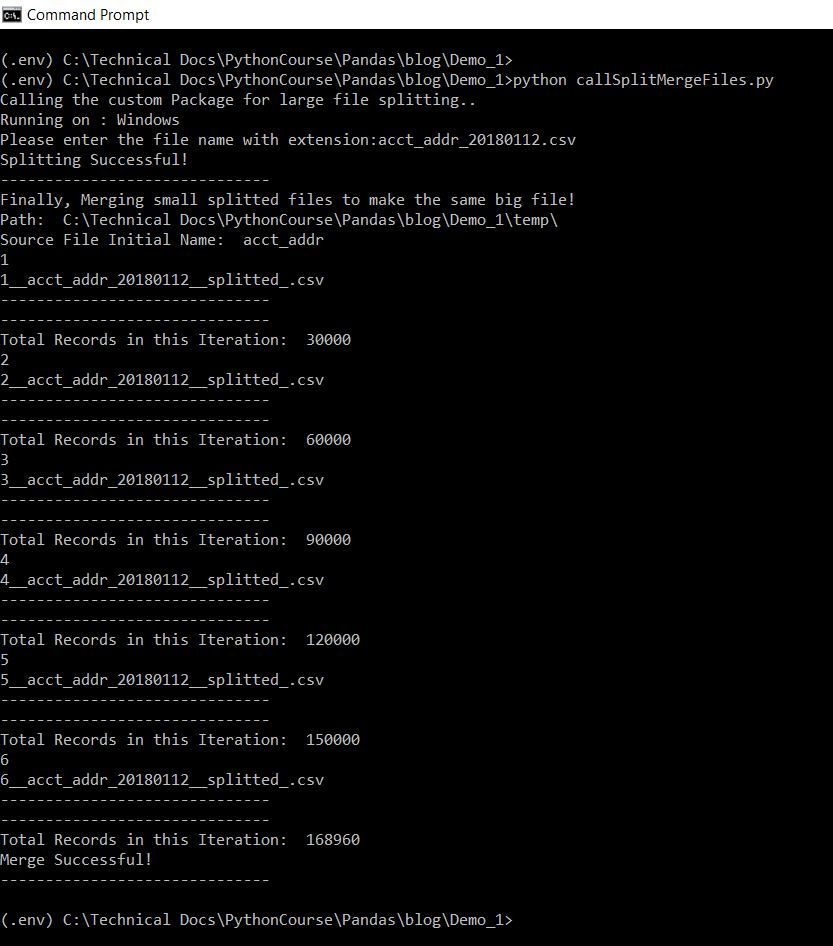##### Mac:And, the final file should look like this –

##### Windows:##### MAC:Left-hand side representing windows final processed/output file, whereas right-hand side representing MAC final processed/output file.

Hope, this will give you some idea about how we can use pandas in various cases apart from conventional data computing.

In this post, I skipped the exception part intentionally. I’ll post one bonus post once my series complete.

Let me know, what do you think.

Till then, Happy Avenging!

Satyaki De

## One thought on “Pandas & Numpy (Space Stone of Programming World)”

1.jbernec says:

Awesome stuff.